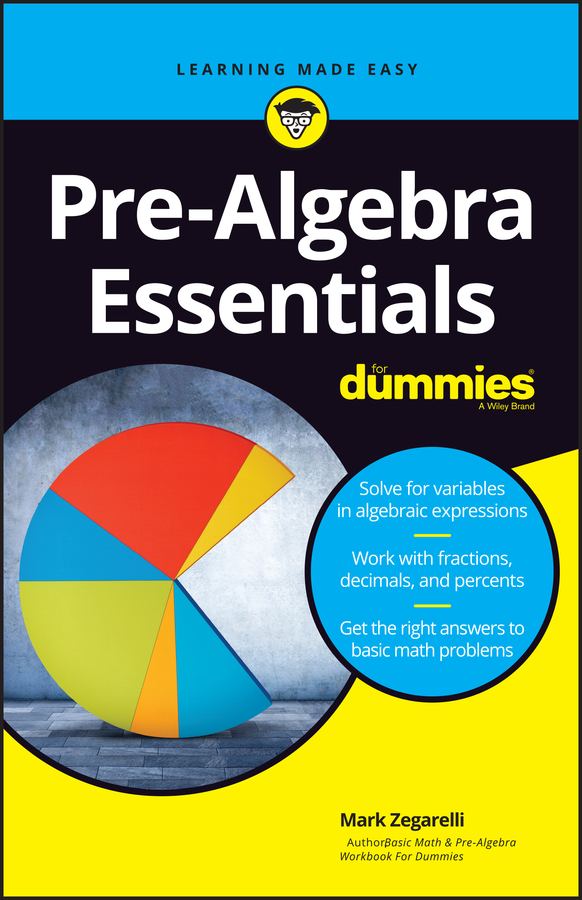##### Pre-Algebra Essentials For DummiesSet theory has four important operations: union, intersection, relative complement, and complement. These operations let you compare sets to determine how they relate to each other.

## Union: Combine elements

The union of two sets is the set of their combined elements. For example, the union of {1, 2} and {3, 4} is {1, 2, 3, 4}. The symbol for this operation is , so

{1, 2} {3, 4} = {1, 2, 3, 4}

Similarly, here’s how to find the union of P and Q:

P Q = {1, 7} {4, 5, 6} = {1, 4, 5, 6, 7}

When two sets have one or more elements in common, these elements appear only once in their union set. For example, consider the union of Q and R. In this case, the elements 4 and 6 are in both sets, but each of these numbers appears once in their union:

Q R = {4, 5, 6} {2, 4, 6, 8, 10} = {2, 4, 5, 6, 8, 10}

The union of any set with itself is itself:

P P = P

Similarly, the union of any set with an empty set, , is itself:

P = P

## Intersection: Find common elements

The intersection of two sets is the set of their common elements (the elements that appear in both sets). For example, the intersection of {1, 2, 3} and {2, 3, 4} is {2, 3}. The symbol for this operation is . You can write the following:

{1, 2, 3} {2, 3, 4} = {2, 3}

Similarly, here’s how to write the intersection of Q and R:

Q R = {4, 5, 6} {2, 4, 6, 8, 10} = {4, 6}

When two sets have no elements in common, their intersection is the empty set ():

P Q = {1, 7} {4, 5, 6} =

The intersection of any set with itself is itself:

P P = P

But the intersection of any set with is :

P =

## Relative complement: Subtract elements

The relative complement of two sets is an operation similar to subtraction. The symbol for this operation is the minus sign (–). Starting with the first set, you remove every element that appears in the second set to arrive at their relative complement. For example,

{1, 2, 3, 4, 5} – {1, 2, 5} = {3, 4}

Similarly, here’s how to find the relative complement of R and Q. Both sets share a 4 and a 6, so you have to remove those elements from R:

R – Q = {2, 4, 6, 8, 10} – {4, 5, 6} = {2, 8, 10}

Note that the reversal of this operation gives you a different result. This time, you remove the shared 4 and 6 from Q:

Q – R = {4, 5, 6} – {2, 4, 6, 8, 10} = {5}

Like subtraction in arithmetic, the relative complement is not a commutative operation. In other words, order is important.

## Complement: Leave elements out

The complement of a set is everything that isn’t in that set. Because everything is a difficult concept to work with, you first have to define what you mean by everything as the universal set (U). For example, suppose you define the universal set like this:

U = {0, 1, 2, 3, 4, 5, 6, 7, 8, 9}

Now, here are a couple of sets to work with:

M = {1, 3, 5, 7, 9}

N = {6}

The complement of each set is the set of every element in U that isn’t in the original set:

U – M = {0, 1, 2, 3, 4, 5, 6, 7, 8, 9} – {1, 3, 5, 7, 9} = {0, 2, 4, 6, 8}

U – N = {0, 1, 2, 3, 4, 5, 6, 7, 8, 9} – {6} = {0, 1, 2, 3, 4, 5, 7, 8, 9}

The complement is closely related to the relative complement. Both operations are similar to subtraction. The main difference is that the complement is always subtraction of a set from U, but the relative complement is subtraction of a set from any other set.

The symbol for the complement is ', so you can write the following:

M' = {0, 2, 4, 6, 8}

N' = {0, 1, 2, 3, 4, 5, 7, 8, 9}